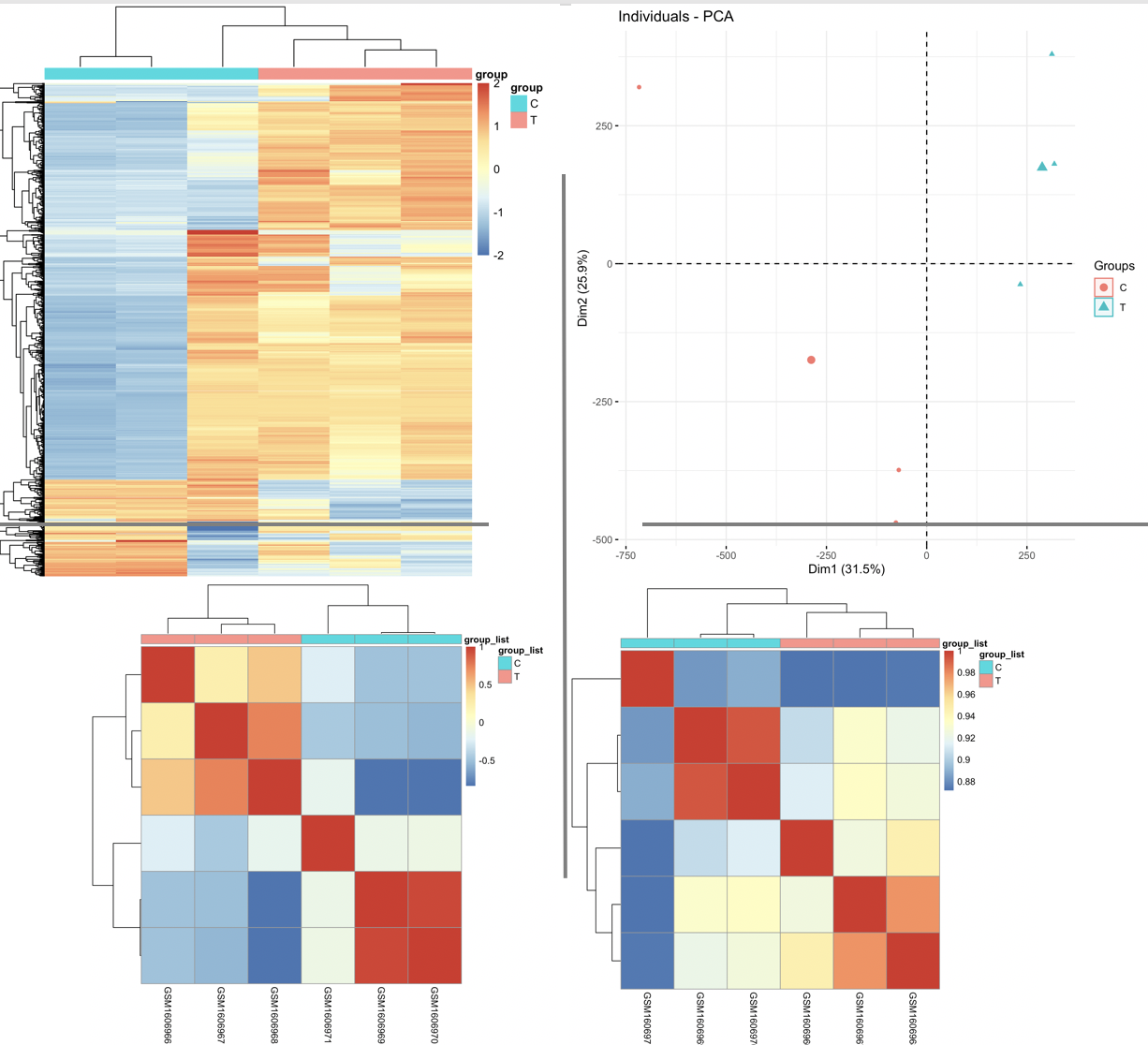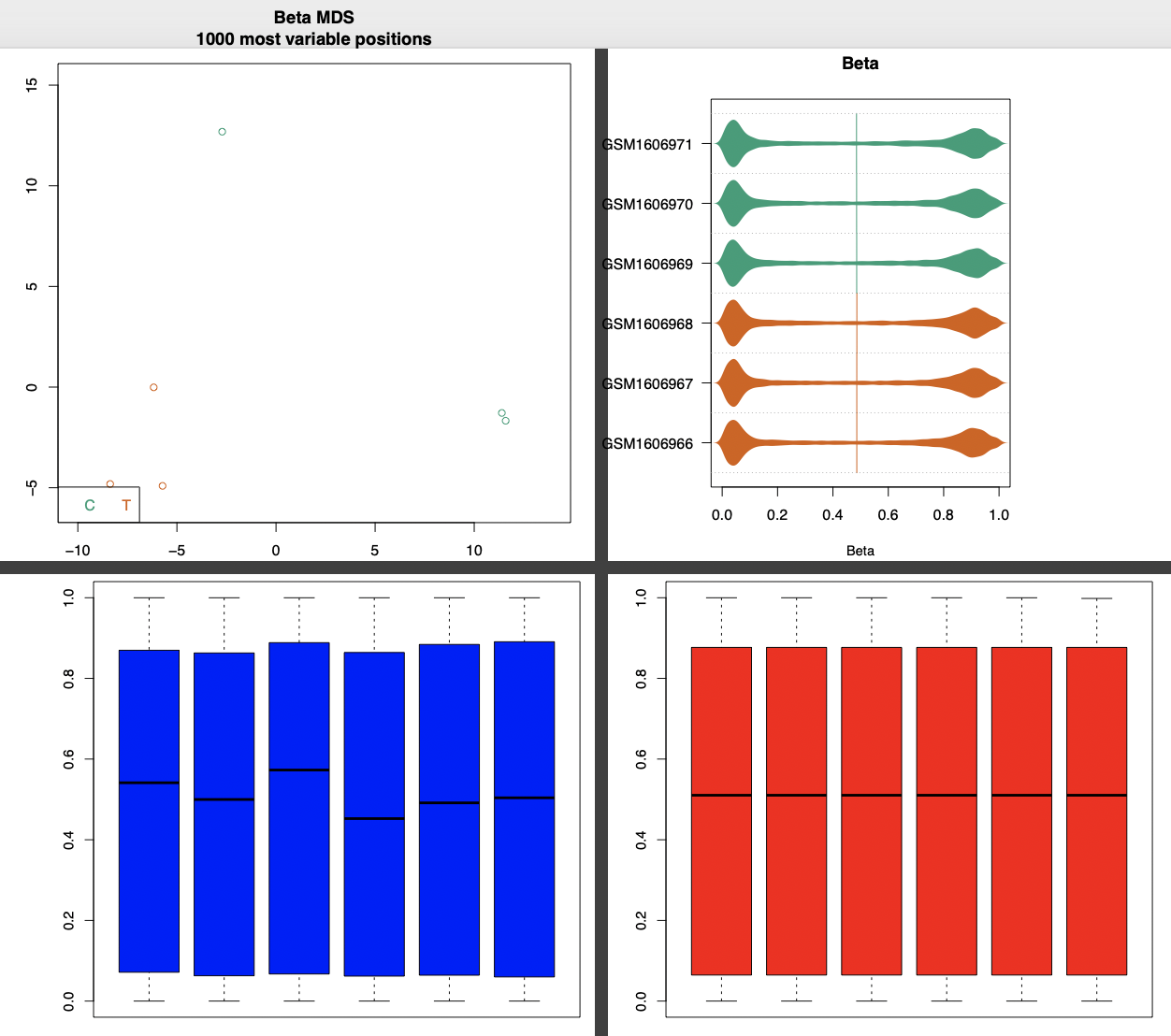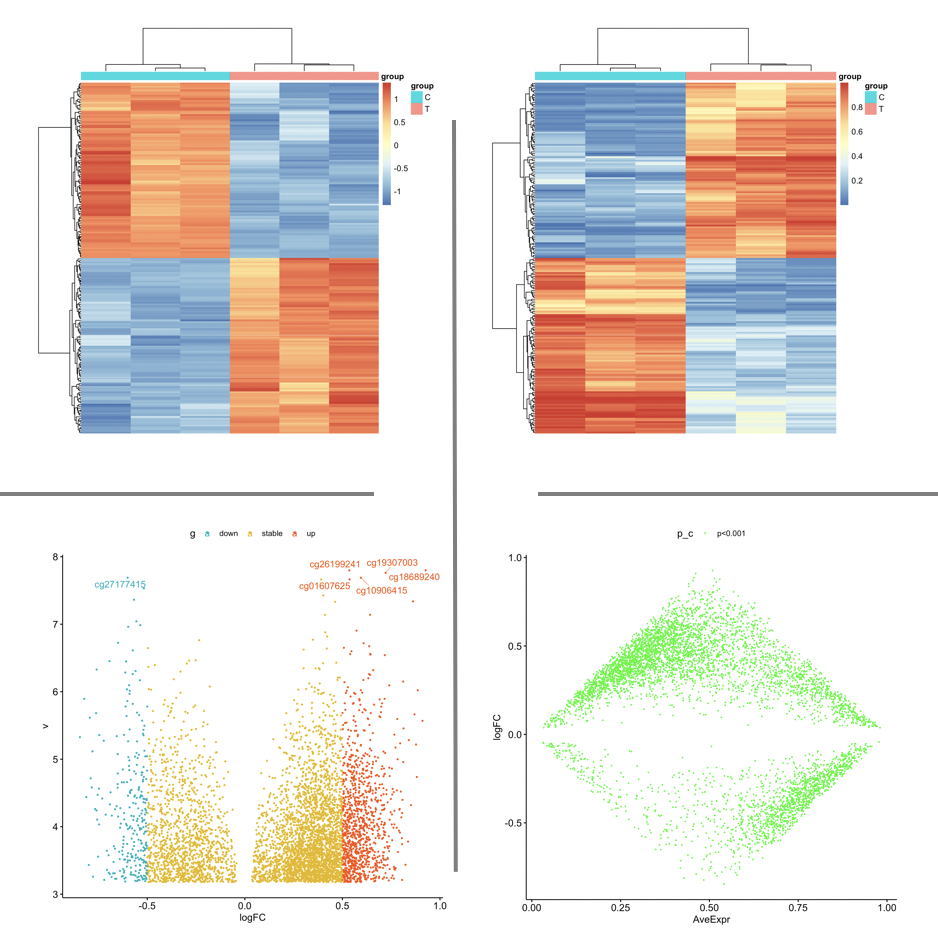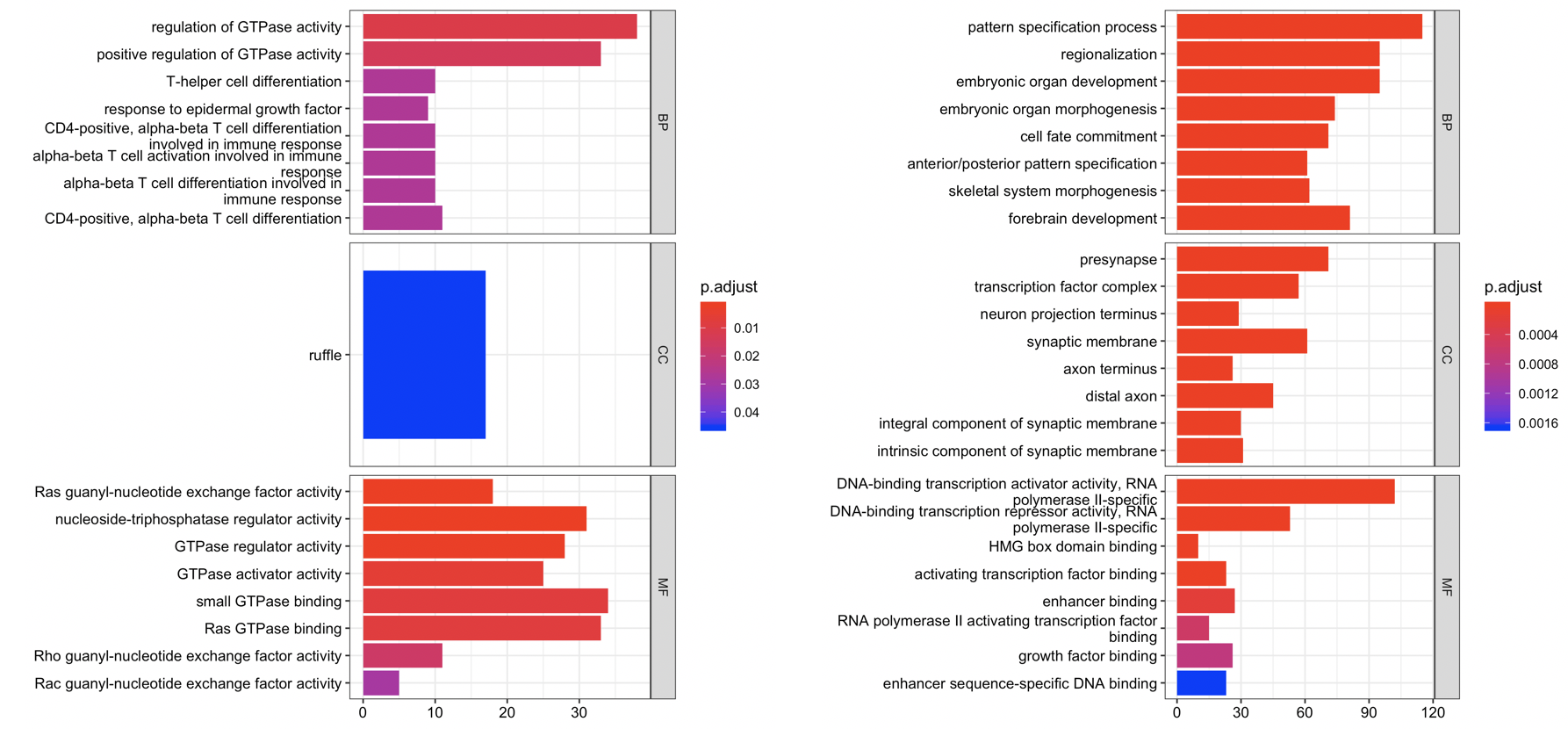# 一个甲基化芯片信号值矩阵差异分析的标准代码

### step0：安装常见的R包

``````#
# 中间肯定会报错，自己机智一点哦
``````

### 如果是idat原始芯片挖掘

#### 如果是甲基化信号值矩阵文件

``````info=read.table("group.txt",sep="\t",header=T)
library(data.table)
# 如果你的甲基化信号矩阵，自己在Excel表格里面整理好。
a[1:4,1:4]
rownames(a)=a[,1]
a=a[,-1]
beta=as.betarix(a)
beta=impute.knn(beta)
a[1:4,1:4]
identical(colnames(a),rownames(b))

library(ChAMP)
# beta 信号值矩阵里面不能有NA值
``````

``````# 如果你使用GEO数据库下载甲基化信号值矩阵文件
# 下面的代码你也需要理解哦。
if(F){
require(GEOquery)
require(Biobase)
eset <- getGEO("GSE68777",destdir = './',AnnotGPL = T,getGPL = F)
beta.m <- exprs(eset[])
## 顺便把临床信息制作一下，下面的代码，具体每一个项目都是需要修改的哦
pD.all <- pData(eset[])
pD <- pD.all[, c("title", "geo_accession", "characteristics_ch1.1", "characteristics_ch1.2")]
names(pD)[c(3,4)] <- c("group", "sex")
pD\$group <- sub("^diagnosis: ", "", pD\$group)
pD\$sex <- sub("^Sex: ", "", pD\$sex)

library(ChAMP)
# beta 信号值矩阵里面不能有NA值
myLoad=champ.filter(beta = beta.m ,pd = pD)
}

# 两种方法，都是为了制作 champ 的对象
``````

### step2 : 甲基化信号值矩阵质量检测### step3：差异分析

``````load(file = 'step1-output.Rdata')
dim(myNorm)
table(group_list)
myDMP <- champ.DMP(beta = myNorm,pheno=group_list)
save(myDMP,file = 'step3-output-myDMP.Rdata')
``````

### step4: 比较champ流程或者minfi流程结果

``````load(file = 'step1-output.Rdata')
# 后续针对 beta.m 进行差异分析, 比如 minfi 包
grset=makeGenomicRatioSetFromMatrix(beta.m,what="Beta")
M = getM(grset)
# 因为甲基化芯片是450K或者850K，几十万行的甲基化位点，统计检验通常很慢。
dmp <- dmpFinder(M, pheno=group_list, type="categorical")
dmpDiff=dmp[(dmp\$qval<0.05) & (is.na(dmp\$qval)==F),]
dim(dmpDiff)

champDiff=myDMP[]

dim(dmpDiff)
dim(champDiff)
length(intersect(rownames(dmpDiff),rownames(champDiff)))
``````### step5：GO或者KEGG等数据库的功能注释